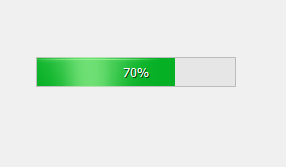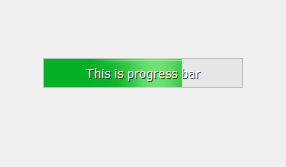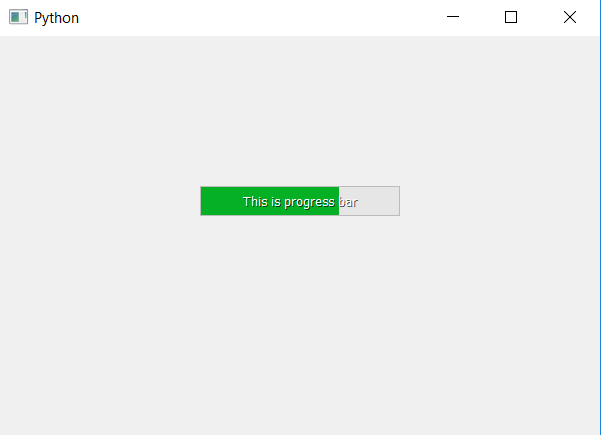# PyQt5 – How to set text to progress bar ?

• Last Updated : 22 Apr, 2020

In this article we will see how to set text to progress bar. When we create a progress bar only progress percentage text is visible but we can also set some text on it.

Below is how normal progress bar vs progress bar with text looks like.In order to do this we will use `setFormat` method, although it is used to set the format i.e percentage indicator but if we pass normal text to it it will display it in process bar.

 `# importing libraries``from` `PyQt5.QtWidgets ``import` `*` `from` `PyQt5 ``import` `QtCore, QtGui``from` `PyQt5.QtGui ``import` `*` `from` `PyQt5.QtCore ``import` `*` `import` `sys`` ` ` ` `class` `Window(QMainWindow):`` ` ` ` `    ``def` `__init__(``self``):``        ``super``().__init__()`` ` `        ``# setting title``        ``self``.setWindowTitle(``"Python "``)`` ` `        ``# setting geometry``        ``self``.setGeometry(``100``, ``100``, ``600``, ``400``)`` ` `        ``# calling method``        ``self``.UiComponents()`` ` `        ``# showing all the widgets``        ``self``.show()`` ` `    ``# method for widgets``    ``def` `UiComponents(``self``):`` ` `        ``# creating progress bar``        ``bar ``=` `QProgressBar(``self``)`` ` `        ``# setting geometry to progress bar``        ``bar.setGeometry(``200``, ``150``, ``200``, ``30``)`` ` `        ``# set value to progress bar``        ``bar.setValue(``70``)`` ` `        ``# setting text ``        ``bar.setFormat(``'This is progress bar'``)`` ` `        ``# setting alignment to centre``        ``bar.setAlignment(Qt.AlignCenter)`` ` ` ` `# create pyqt5 app``App ``=` `QApplication(sys.argv)`` ` `# create the instance of our Window``window ``=` `Window()`` ` `# start the app``sys.exit(App.``exec``())`

Output :My Personal Notes arrow_drop_up